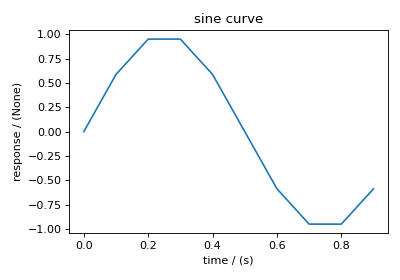# Getting started with csdmpy package¶

We have put together a set of guidelines for importing the csdmpy package and related methods and attributes. We encourage the users to follow these guidelines to promote consistency, amongst others. Import the package using

>>> import csdmpy as cp


To load a .csdf or a .csdfe file, use the load() method of the csdmpy module. In the following example, we load a sample test file.

>>> filename = cp.tests.test01 # replace this with your file's name.


Here, testdata1 is an instance of the CSDM class.

At the root level, the CSDM object includes various useful optional attributes that may contain additional information about the dataset. One such useful attribute is the description key, which briefs the end-users on the contents of the dataset. To access the value of this attribute use,

>>> testdata1.description
'A simulated sine curve.'


## Accessing dimensions and dependent variables of the dataset¶

An instance of the CSDM object may include multiple dimensions and dependent variables. Collectively, the dimensions form a multi-dimensional grid system, and the dependent variables populate this grid. In csdmpy, dimensions and dependent variables are structured as list object. To access these lists, use the dimensions and dependent_variables attribute of the CSDM object, respectively. For example,

>>> x = testdata1.dimensions
>>> y = testdata1.dependent_variables


In this example, the dataset contains one dimension and one dependent variable.

You may access the instances of individual dimension and dependent variable by using the proper indexing. For example, the dimension and dependent variable at index 0 may be accessed using x and y, respectively.

Every instance of the Dimension object has its own set of attributes that further describe the respective dimension. For example, a Dimension object may have an optional description attribute,

>>> x.description
'A temporal dimension.'


Similarly, every instance of the DependentVariable object has its own set of attributes. In this example, the description attribute from the dependent variable is

>>> y.description
'A response dependent variable.'


### Coordinates along the dimension¶

Every dimension object contains a list of coordinates associated with every grid index along the dimension. To access these coordinates, use the coordinates attribute of the respective Dimension instance. In this example, the coordinates are

>>> x.coordinates
<Quantity [0. , 0.1, 0.2, 0.3, 0.4, 0.5, 0.6, 0.7, 0.8, 0.9] s>


Note

x.coordinates returns a Quantity instance from the Astropy package. The csdmpy module utilizes the units library from astropy.units module to handle physical quantities. The numerical value and the unit of the physical quantities are accessed through the Quantity instance, using the value and the unit attributes, respectively. Please refer to the astropy.units documentation for details. In the csdmpy module, the Quantity.value is a Numpy array. For instance, in the above example, the underlying Numpy array from the coordinates attribute is accessed as

>>> x.coordinates.value
array([0. , 0.1, 0.2, 0.3, 0.4, 0.5, 0.6, 0.7, 0.8, 0.9])


### Components of the dependent variable¶

Every dependent variable object has at least one component. The number of components of the dependent variable is determined from the quantity_type attribute of the dependent variable object. For example, a scalar quantity has one-component, while a vector quantity may have multiple components. To access the components of the dependent variable, use the components attribute of the respective DependentVariable instance. For example,

>>> y.components
array([[ 0.0000000e+00,  5.8778524e-01,  9.5105654e-01,  9.5105654e-01,
5.8778524e-01,  1.2246469e-16, -5.8778524e-01, -9.5105654e-01,
-9.5105654e-01, -5.8778524e-01]], dtype=float32)


The components attribute is a Numpy array. Note, the number of dimensions of this array is $$d+1$$, where $$d$$ is the number of Dimension objects from the dimensions attribute. The additional dimension in the Numpy array corresponds to the number of components of the dependent variable. For instance, in this example, there is a single dimension, i.e., $$d=1$$ and, therefore, the value of the components attribute holds a two-dimensional Numpy array of shape

>>> y.components.shape
(1, 10)


where the first element of the shape tuple, 1, is the number of components of the dependent variable and the second element, 10, is the number of points along the dimension, i.e., x.coordinates.

## Plotting the dataset¶

It is always helpful to represent a scientific dataset with visual aids such as a plot or a figure instead of columns of numbers. As such, throughout this documentation, we provide a figure or two for every example dataset. We make use of Python’s Matplotlib library for generating these figures. The users may, however, use their favorite plotting library.

The following snippet plots the dataset from this example. Here, the axis_label is an attribute of both Dimension and DependentVariable instances, and the name is an attribute of the DependentVariable instance.

>>> import matplotlib.pyplot as plt

>>> plt.figure(figsize=(5, 3.5))
>>> plt.plot(x.coordinates, y.components)
>>> plt.xlabel(x.axis_label)
>>> plt.ylabel(y.axis_label)
>>> plt.title(y.name)
>>> plt.tight_layout()
>>> plt.show()